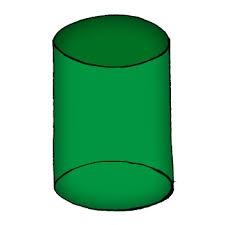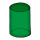# The cylinder

The cylinder has a surface area of 300 square meters, while the cylinder's height is 12 m. Calculate the volume of this cylinder.

V =  374.3807 m3

### Step-by-step explanation:Did you find an error or inaccuracy? Feel free to write us. Thank you!Tips to related online calculators
Looking for help with calculating roots of a quadratic equation?
Do you have a linear equation or system of equations and looking for its solution? Or do you have a quadratic equation?
Tip: Our volume units converter will help you with the conversion of volume units.

## Related math problems and questions:

• Shell area cyThe cylinder has a shell content of 300 cm square, while the height of the cylinder is 12 cm. Calculate the volume of this cylinder.
• CylinderThe cylinder surface is 922 dm2. Its height is equal to the radius of the base. Calculate the height of this cylinder.
• Volume and surfaceCalculate the volume and surface area of the cylinder when the cylinder height and base diameter is in a ratio of 3:4 and the area of the cylinder jacket is 24 dm2.
• The cylinderIn a rotating cylinder it is given: the surface of the shell (without bases) S = 96 cm2 and the volume V = 192 cm cubic. Calculate the radius and height of this cylinder.
• Diameter = heightThe cylinder's surface, the height of which is equal to the diameter of the base, is 4239 cm square. Calculate the cylinder volume.
• Equilateral cylinderEquilateral cylinder (height = base diameter; h = 2r) has a volume of V = 199 cm3 . Calculate the surface area of the cylinder.
• Height as diameter of baseThe rotary cylinder has a height equal to the base diameter and a surface of 471 cm2. Calculate the volume of a cylinder.
• The blockThe block, the edges formed by three consecutive GP members, has a surface area of 112 cm2. The sum of the edges that pass through one vertex is 14 cm. Calculate the volume of this block.
• The cubeThe cube has a surface area of 486 m ^ 2. Calculate its volume.
• Rotary cylinderIn the rotary cylinder it is given: surface S = 96 cm2 and volume V = 192 cm cubic. Calculate its radius and height.
• The surfaceThe surface of the cylinder is 1570 cm2, its height is 15 cm. Find its volume and radius of the base.
• Regular square prismThe volume of a regular square prism is 192 cm3. The size of its base edge and the body height is 1: 3. Calculate the surface of the prism.
• Hexagonal prism 2The regular hexagonal prism has a surface of 140 cm2 and height of 5 cm. Calculate its volume.
• Hard cone problemThe surface of the cone is 200 cm², its height is 7 centimeters. Calculate the volume of this cone.
• The ballThe ball has a radius of 2m. What percentage of the surface and volume is another sphere whose radius is 20% larger?
• Surface of the cylinderCalculate the surface of the cylinder for which the shell area is Spl = 20 cm2 and the height v = 3.5 cm
• The poolThe cube-shaped pool has 140 cubic meters of water. Determine the dimensions of the bottom if the depth of the water is 200 cm and one dimension of the base is 3 m greater than the other. What are the dimensions of the pool bottom?